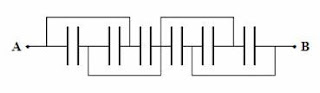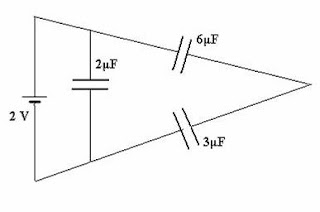## Saturday, July 09, 2011

### Karnataka CET Multiple Choice Questions on Combination of Capacitors

The following question appeared in Karnataka Common Entrance Test (CET) 2010 question paper. Even though at the first reading it may appear somewhat difficult for you on seeing the circuit, a careful look at the circuit will assure you that it’s simple.All capacitors used in the diagram are identical and each is of capacitance C. Then the effective capacitance between the points A and B is

(a) 1.5 C

(b) 6 C

(c) C

(d) 3 C

The first three capacitors are in parallel, giving an effective capacitance 3 C. The last three capacitors also are in parallel, giving an effective capacitance 3 C.. These two parallel combinations are connected in series. Therefore, the effective capacitance between the points A and B is 3 C/2 = 1.5 C.

The following question appeared in the Karnataka CET 2008 question paper:

How many 6 μF, 200 V condensers are needed to make a condenser of 18 μF, 600 V?

(1) 9

(2) 18

(3) 3

(4) 27

Since the voltage rating of each 6 μF capacitor is 200 V, you should connect 3 capacitors in series to obtain the required voltage rating of 600 V. But then the effective capacitance of the series combination of these three capacitors will be 2 μF (6/3 = 2). To obtain the required capacitance of 18 μF, you need to connect nine such series combinations in parallel. So the total number of capacitors required is 9×3 = 27.The following question also appeared in the Karnataka CET 2008 question paper:

The total energy stored in the condenser system shown in the figure will be

(1) 2 μJ

(2) 4 μJ

(3) 8 μJ

(4) 16 μJ

The series combination of 6 μF and 3 μF gives an effective capacitance of (6×3)/(6+3) = 2 μF. Since this is in parallel with a 2 μF capacitor, the effective capacitance C across the 2 volt battery is 4 μF. The energy stored in the system of capacitors is ½ CV2 = (½)×(4×10–6) ×22 = 8×10–6 J = 8 μJ.

You will find a few more multiple choice questions (with solution) in this section here.

#### 1 comment:

1.hv film capacitor manufacturer Thanks for a very interesting blog. What else may I get that kind of info written in such a perfect approach? I’ve a undertaking that I am simply now operating on, and I have been at the look out for such info.